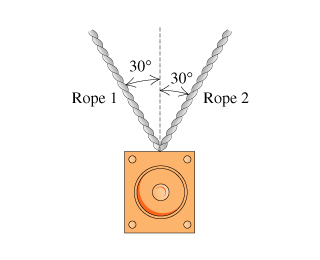# Problem: (A) A 20-kg loudspeaker is suspended 2.0 m below the ceiling by two ropes that are each 30° from vertical.Find the value of T, the magnitude of the tension in either of the ropes.Express your answer in newtons.T=(B) Suppose that the loudspeaker in the problem had a mass of 500 kg and the ropes hung 20° from the vertical. Into which of the following intervals would you expect T to fall? You do not have to calculate T exactly to answer this question; just make an estimate.a) 500 to 1000 Nb) 1000 to 2000 Nc) 2000 to 4000 Nd) 4000 to 8000 Ne) 8000 to 16,000 N

###### FREE Expert Solution

Newton's second law:

$\overline{){\mathbf{F}}{\mathbf{=}}{\mathbf{m}}{\mathbf{a}}}$

(a)

Let the x-axis pint to the left and the y-axis upward.

The weight points downwards.

The component of the tension, FTy, points opposite to the weight, thus making the adjacent side.

cos θ = adjacent/hypotenuse = FTy/FT

FTy = FT cos θ

86% (102 ratings)###### Problem Details

(A) A 20-kg loudspeaker is suspended 2.0 m below the ceiling by two ropes that are each 30° from vertical.Find the value of T, the magnitude of the tension in either of the ropes.

T=

(B) Suppose that the loudspeaker in the problem had a mass of 500 kg and the ropes hung 20° from the vertical. Into which of the following intervals would you expect T to fall? You do not have to calculate exactly to answer this question; just make an estimate.

a) 500 to 1000 N

b) 1000 to 2000 N

c) 2000 to 4000 N

d) 4000 to 8000 N

e) 8000 to 16,000 N